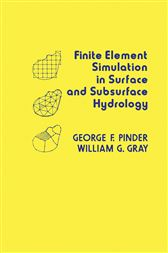Finite Element Simulation in Surface and Subsurface HydrologyUS\$72.95
ISBNs
• 9780125569507
• 9781483270425
Finite Element Simulation in Surface and Subsurface Hydrology provides an introduction to the finite element method and how the method is applied to problems in surface and subsurface hydrology.
The book presents the basic concepts of the numerical methods and the finite element approach; applications to problems on groundwater flow and mass and energy transport; and applications to problems that involve surface water dynamics. Computational methods for the solution of differential equations; classification of partial differential equations; finite difference and weighted residual integral techniques; and The Galerkin finite element method are discussed as well.
The text will be of value to engineers, hydrologists, and students in the field of engineering.

• Elsevier Science; September 2013
• ISBN: 9781483270425